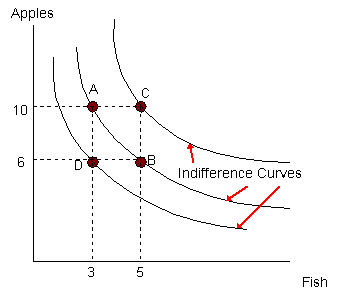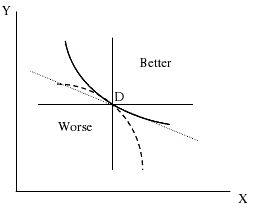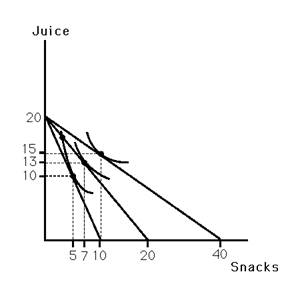### Why should I choose AnalystNotes?

AnalystNotes specializes in helping candidates pass. Period.

### Subject 2. Indifference Curves

An indifference curve shows the combination of two products that provide an individual with a given level of utility (satisfaction). It is a curve, convex from below, that separates the consumption bundles that are more preferred by an individual from those that are less preferred. The points on the curve represent combinations of goods that are equally preferred by the individual. For example, the bundle at point A of 10 apples and 3 fish provides the same satisfaction as the bundle at point B of 6 apples and 5 fish.Indifference curves provide a diagrammatic picture of how an individual ranks alternative consumption bundles.

• More goods are preferable to fewer goods. Thus, bundles on indifference curves lying farthest to the northeast of a diagram are always preferred. For example, the bundle at C is clearly preferred to those points along the indifference curve and the bundle at point D is clearly inferior to those points along the curve.

• Goods are substitutable. Therefore, indifference curves slope downward to the right.

• The value of a good declines as it is consumed more intensively. Therefore, indifference curves are always convex when viewed from below. The slope of the indifference curve is equal to the marginal rate of substitution: the amount of one good that is just sufficient to compensate the consumer for the loss of a unit of the other good.

• Indifference curves are everywhere dense. That is, an indifference curve can be drawn through any point on the diagram; any two bundles of goods can be compared by the individual.

• Indifference curves for one consumer cannot cross. If they did, rational ordering would be violated and the postulate that more goods are better than fewer goods would be violated.

If two consumers have different marginal rates of substitution, they can both benefit from the voluntary exchange of one good for the other.

#### Practice Question 1

The slope of an indifference curve is always negative. This characteristic comes from ______.

A. the axiom of completeness
B. the axiom of transitivity
C. the axiom of nonsatiation

That is, "the more, the better" applies to comparing different baskets of commodities. If we randomly spot a certain point on the diagram representing two goods, we will see that any combinations of these two goods that lie in the northeast of this point are better; symmetrically, any combinations lying in the southwest of this point are less desired. So, if we connect all dots representing the same level of utility as point A and form a curve, the curve should go from northwest to southeast.#### Practice Question 2

Which statement is true?

A. The absolute value of the slope of the indifference curve represents the marginal rate of substitution (MRS).
B. The utility theory assumes that all consumers should have the same preference.
C. Two indifference curves for two different consumers cannot intersect.

A is true. While the slope of indifference curve is always negative, MRS is always a positive value. B is false: consumers can have different preferences. C is also false. Yes, two indifference curves can intersect. However, two indifference curves for one single consumer can never intersect.

#### Practice Question 3

There is an infinite number of indifference curves which cover the whole area of the axiom panel. This comes from ______.

A. the axiom of completeness
B. the axiom of transitivity
C. the axiom of nonsatiation

Obviously, this relates to the axiom of completeness. Since an individual can rank any of the baskets of goods and every point on the axiom panel represents one particular basket of goods, then every point should have one and only one indifference curve going through it.

#### Practice Question 4

The following figure shows Bobby's indifference map for juice and snacks. Also shown are three budget lines resulting from different prices for snacks, assuming he has \$20 to spend on these goods. Which of the following points are on Bobby's price-consumption curve?A. 10 snacks and 20 juices
B. 10 snacks and 15 juices
C. 10 snacks and 5 juices

#### Practice Question 5

Indifference curves for one consumer never intersect. This comes from ______.

A. the axiom of completeness
B. the axiom of transitivity
C. the axiom of nonsatiation

Suppose two indifference curves intersect at point A. We can find two points, one on each indifference curve, with same amount of good X, but C has more of good Y than B. Since A and B are on the same indifference curve, we have A = B and at the same time we also have A = C. Applying the axiom of transitivity, we can immediately see that B = C should hold. But from the weak version of the axiom of nonsatiation, we also have C > B. Thus, a conflict appears. So, indifference curves can never intersect with each other.#### Practice Question 6

The amount of one good that is just sufficient to compensate the consumer for the loss of some amount of another good is called ______.

A. the marginal rate of substitution
B. consumer surplus
C. the substitution effect

The marginal rate of substitution is the slope of the indifference curve and represents the amount of good x that the consumer must be compensated with in order to reduce his or her consumption of good y by one unit.

#### Practice Question 7

Evaluate the following statements:

I. All points that lie above (to the northeast of) an indifference curve represent consumption bundles preferred to those represented by all points on that curve.
II. The indifference curves of a rational decision-maker cannot cross.

A. Both statements are true.
B. I is false; II is true.
C. I is true; II is false.

Both I and II are true because the indifference curve represents consumption bundles that give the consumer exactly the same level of satisfaction. Since it is assumed that goods are normal (that is, that more of the good is always better and preferred to less of the good), then bundles which lie further from the origin than those on the indifference curve are preferred. II is true because intersecting indifference curves imply that the consumer is not rational: it is not clear if he or she prefers more goods to less goods, since it is unclear which indifference curve gives him or her more satisfaction.

#### Practice Question 8

The characteristics of indifference curves do not include which of the following?

A. Indifference curves are everywhere dense and cannot cross.
B. Indifference curves lying farthest to the northeast of a diagram are always preferred.
C. Indifference curves are always concave from below.

Indifference curves are always convex from below, not concave. The other characteristics assure us that all bundles of goods can be compared, that a bundle with less is not viewed indifferently when compared to a bundle with more, and that goods are substitutable at a decreasing rate.

#### Practice Question 9

As a consumer moves along an indifference curve, ______

A. prices are held constant, but income changes.
B. prices and income are held constant, but total utility changes.
C. total utility is held constant, but prices and income change.

An indifference curve is a curve that shows combinations of goods to which an individual is indifferent. That is, total utility remains constant.

#### Practice Question 10

When the price of a good changes, the substitution effect can be found by comparing the equilibrium quantities purchased ______.

A. on the old budget line and the new budget line
B. on the original indifference curve when faced with the original prices and when faced with the new prices
C. on the new budget line and a hypothetical budget line that is a parallel shift back to the original indifference curve